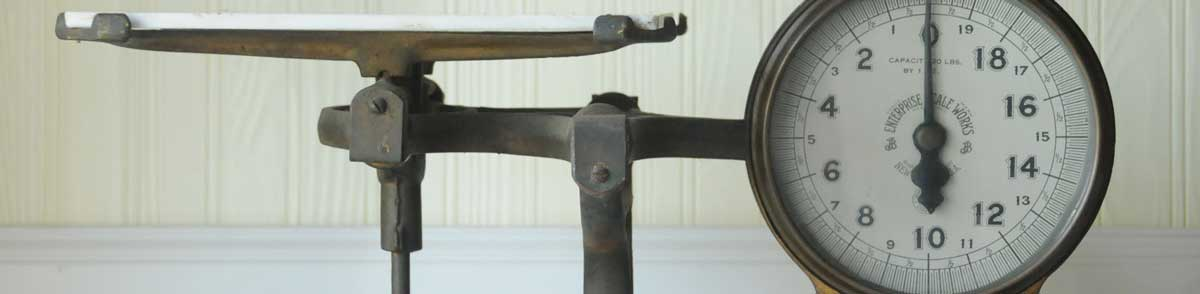# Milligrams to Ounces Conversion

Enter the weight in milligrams below to get the value converted to ounces.

Results in Ounces:1 mg = 3.5274E-5 oz
Hint: use a scientific notation calculator to convert E notation to decimal

## How to Convert Milligrams to OuncesTo convert a milligram measurement to an ounce measurement, multiply the weight by the conversion ratio.

Since one milligram is equal to 3.5274E-5 ounces, you can use this simple formula to convert:

ounces = milligrams × 3.5274E-5

The weight in ounces is equal to the milligrams multiplied by 3.5274E-5.

For example, here's how to convert 50,000 milligrams to ounces using the formula above.
50,000 mg = (50,000 × 3.5274E-5) = 1.763698 oz

## Milligrams

The milligram is the mass equal to 1/1,000 of a gram, or 0.0154 grains.

The milligram, or milligramme, is an SI unit of weight in the metric system. In the metric system, "milli" is the prefix for 10-3. Milligrams can be abbreviated as mg; for example, 1 milligram can be written as 1 mg.

## Ounces

One ounce is a unit of mass/weight equal to 1/16 of a pound. The common ounce should not be confused with the troy ounce, which is equal to 1/12 of a troy pound.

The ounce is a US customary and imperial unit of weight. Ounces can be abbreviated as oz; for example, 1 ounce can be written as 1 oz.

## Milligram to Ounce Conversion Table

Milligram measurements converted to ounces
Milligrams Ounces
1 mg 0.000035274 oz
2 mg 0.000070548 oz
3 mg 0.000106 oz
4 mg 0.000141 oz
5 mg 0.000176 oz
6 mg 0.000212 oz
7 mg 0.000247 oz
8 mg 0.000282 oz
9 mg 0.000317 oz
10 mg 0.000353 oz
100 mg 0.003527 oz
1,000 mg 0.035274 oz
10,000 mg 0.35274 oz
100,000 mg 3.5274 oz

## References

1. James Bucki, Troy Ounce vs. Ounce, The Spruce, https://www.thesprucecrafts.com/troy-ounce-vs-ounce-4588158